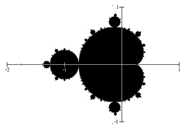## Introduction to Fractals Generated by Complex Numbers

You may be familiar with the fractal in the image below.  The boundary of this shape exhibits quasi-self-similarity, in that portions look very similar to the whole. This object is called the Mandelbrot set and is generated by iterating a simple recursive rule using complex numbers. In this lesson, you will first learn about the arithmetic of complex numbers so you can understand how a fractal like the Mandelbrot set is generated.